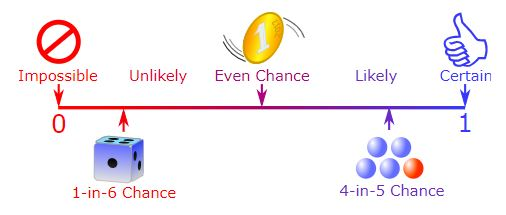### Probability and Statistics 2020/21

This course is designed to provide the mathematical foundation needed by engineering students at the undergraduate level in statistics and probability. The mathematics covered in this course will enable students to solve mathematical problems in statistics and probability that arise in engineering at the undergraduate level and that practicing engineers are likely to encounter in industry.